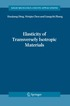Fler böcker inom
Format
Språk
Engelska
Antal sidor
192
Utgivningsdatum
2011-10-08
Upplaga
Softcover reprint of the original 1st ed. 1983
Förlag
Springer
Illustrationer
XII, 192 p.
Dimensioner
234 x 156 x 11 mm
Vikt
300 g
Antal komponenter
1
Komponenter
1 Paperback / softback
ISBN
9789400968684

### Du kanske gillar# Elastic wave propagation in transversely isotropic media

1059
Skickas inom 10-15 vardagar.
Fri frakt inom Sverige för privatpersoner.
Finns även som
789
• Skickas inom 11-20 vardagar (specialorder)
899
In this monograph I record those parts of the theory of transverse isotropic elastic wave propagation which lend themselves to an exact treatment, within the framework of linear theory. Emphasis is placed on transient wave motion problems in two- and three-dimensional unbounded and semibounded solids for which explicit results can be obtained, without resort to approximate methods of integration. The mathematical techniques used, many of which appear here in book form for the first time, will be of interest to applied mathematicians, engeneers and scientists whose specialty includes crystal acoustics, crystal optics, magnetogasdynamics, dislocation theory, seismology and fibre wound composites. My interest in the subject of anisotropic wave motion had its origin in the study of small deformations superposed on large deformations of elastic solids. By varying the initial stretch in a homogeneously deformed solid, it is possible to synthesize aniso tropic materials whose elastic parameters vary continuously. The range of the parameter variation is limited by stability considerations in the case of small deformations super posed on large deformation problems and (what is essentially the same thing) by the of hyperbolicity (solids whose parameters allow wave motion) for anisotropic notion solids. The full implication of hyperbolicity for anisotropic elastic solids has never been previously examined, and even now the constraints which it imposes on the elasticity constants have only been examined for the class of transversely isotropic (hexagonal crystals) materials.

## Passar bra ihop

1.2. +
3.De som köpt den här boken har ofta också köpt Elasticity of Transversely Isotropic Materials av Haojiang Ding, Weiqiu Chen, Ling Zhang (inbunden).

## Kundrecensioner

Har du läst boken? Sätt ditt betyg »

## Innehållsförteckning

1. Basic equations.- 1. The Linearized Equations of Motion of an Anisotropic Elastic Solid.- 2. The Effect on the Equations of Motion of a Coordinate Rotation.- 3. The Elasticities for a Transversely Isotropic Solid.- 4. The Constraints on the c??'s of Positive Definiteness.- 5. The Constraints on the c??'s of Strong Ellipticity.- 6. The Uncoupled Equations of Motion in Two-Dimensions.- 7. The Uncoupled Equations of Motion in Three-Dimensions.- 8. Some Other Transversely Isotropic Continuum Theories.- 2. Wave front shape caused by a point source in unbounded media.- I Two Space Dimensions.- 1. The Characteristic Partial Differential Equation for the Wave Front.- 2. The Normal Curve.- 3. Bitangents and the Existence of Inflection Points on the Normal Curve.- 4. Bitangents Which Cross Both Coordinate Axes of the Normal Curve.- 5. Double Points of the Normal Curve.- 6. Classification of the Normal Curve Shape.- 7. Solution of the Characteristic Partial Differential Equation.- 8. Wave Front Construction as Envelope of Line Waves.- 9. Classification of the Wave Front Curve when Q is Strictly Hyperbolic.- 10. Normal and Wave Front Curves for some Hexagonal Materials.- 11. Wave Front Construction When the Normal Curve has Double Points - Convex Hull.- 12. Necessary Condition for the Existence of Wave Front Lids.- 13. The Wave Front Shape When the Normal Curve has Double Points - Conclusion.- 14. Remarks on the Wave Front Shape When the Line Load is Not Perpendicular to the Material Symmetry Axis.- II Three Space Dimensions.- 15. The Characteristic Partial Differential Equation for the Wave Front.- 16. The Normal Surface-Rotational Symmetry.- 17. Multiple Points of the Normal Surface.- 18. Wave Front Construction as Envelope of Plane Waves.- 19. The Existence of Wave Front Lids.- 20. Normal and Wave Surfaces for some Hexagonal Materials.- 3. Green's tensor for the displacement field in unbounded media.- I Two Space Dimensions.- 1. Integral Transform Representation of the Displacement Field.- 2. Transform Inversion and Reduction to a Residue Calculation.- 3. The Existence of Lacunas.- 4. Examination of the Complex p-Roots of Q(1, p, y? + z?p) = 0 When the Observation Point is on a Coordinate Axis.- 5. Displacement Components Along the Symmetry Axis for Some Hexagonal Materials.- 6. The Solution Behaviour Near the Wave Front.- 7. The Stress Field Induced by a Line Load.- 8. Remarks on the Green's Tensor Representation When the Line Load is Not Perpendicular to the Material Symmetry Axis.- II Three Space Dimensions.- 9. Integral Transform Representation of the Displacement Field.- 10. Transform Inversion for the Special Case ? = ? + ?.- 11. Transform Inversion for the General Case Along the Symmetry Axis.- 12. Application to Some Hexagonal Crystals.- 13. Herglotz-Petrowski Formula for the Displacement Field as an Integral Over the Slowness Surface.- 14. Differential Geometry of the Slowness Surface N.- 15. Remarks Concerning the Displacement Behaviour Near the Wave Front.- 4. Surface motion of a two-dimensional half-space (Lamb's problem).- 1. Formulation of the Problem.- 2. Integral Transform Representation of the Solution When the Fourier Inversion Path is Free of Branch Points.- 3. Transform Inversion for Materials Satisfying Condition (1) of Table 11.- 4. Transform Inversion for Materials Satisfying Condition (2) of Table 11.- 5. Transform Inversion for Materials Satisfying Condition (3) of Table 11.- 6. Graph of the Surface Displacements for Some Hexagonal Crystals.- 5. Epicenter and epicentral-axis motion of a three-dimensional half-space.- 1. Problem Formulation for the Epicenter Motion of a Half-Space Due to the Sudden Application of a Buried Point Source.- 2. Transform Inversion at the Epicenter for Materials Satisfying Condition (1) of Table 11.- 3. Discussion of the ?-Plane Branch Points and the Cagniard Path When the Real ?-Axis is Not Free of Branch Points.- 4. Transform Inversion at the Epicenter for Materials Satisfying Co